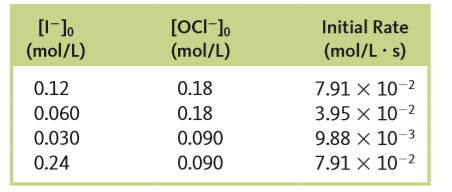# Problem: The reactionI - (aq) + OCl - (aq) → IO - (aq) + Cl - (aq)was studied, and the following data were obtained:c. Calculate the initial rate for an experiment where both I  - and OCl - are initially present at 0.15 mol/L.

###### FREE Expert Solution
84% (296 ratings)
###### FREE Expert Solution

We are asked to calculate the initial rate for an experiment where both I  - and OCl - are initially present at 0.15 mol/L

To do so, we shall follow these steps:

Step 1Obtain the rate law equation.

Step 2: Determine the rate constant, k.

Step 3Determine the initial rate.

84% (296 ratings)###### Problem Details

The reaction

I - (aq) + OCl - (aq) → IO - (aq) + Cl - (aq)

was studied, and the following data were obtained:c. Calculate the initial rate for an experiment where both I  - and OCl - are initially present at 0.15 mol/L.

What scientific concept do you need to know in order to solve this problem?

Our tutors have indicated that to solve this problem you will need to apply the Rate Law concept. You can view video lessons to learn Rate Law. Or if you need more Rate Law practice, you can also practice Rate Law practice problems.

What is the difficulty of this problem?

Our tutors rated the difficulty ofThe reactionI - (aq) + OCl - (aq) → IO - (aq) + Cl - (aq)was...as high difficulty.

How long does this problem take to solve?

Our expert Chemistry tutor, Dasha took 11 minutes and 27 seconds to solve this problem. You can follow their steps in the video explanation above.

What professor is this problem relevant for?

Based on our data, we think this problem is relevant for Professor Bernskoetter's class at MIZZOU.

What textbook is this problem found in?

Our data indicates that this problem or a close variation was asked in Chemistry: An Atoms First Approach - Zumdahl Atoms 1st 2nd Edition. You can also practice Chemistry: An Atoms First Approach - Zumdahl Atoms 1st 2nd Edition practice problems.